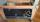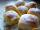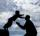Jan and Dan

Jan and Dan had the same money. Jan bought 5 workbooks and left him 15 CZK. Dan 6 and left him nothing. How much money have in total?

Result

x =  180 Kc

Solution:Leave us a comment of this math problem and its solution (i.e. if it is still somewhat unclear...):Be the first to comment!To solve this verbal math problem are needed these knowledge from mathematics:

Do you have a linear equation or system of equations and looking for its solution? Or do you have quadratic equation?

Next similar math problems:

1. Seven timesWhich number seven times is just as higher as 27, how much is smaller than 29?
2. Cakes1/3 poppy cake, 1/3 apple, 15 pieces of cheese. How many are totally cakes?
3. Eq1Solve equation: 4(a-3)=3(2a-5)
4. Six te 2If 3t-7=5t, then 6t=
5. If-then equationIf 5x - 17 = -x + 7, then x =
6. Forest nurseryIn the forest nursery after winter, they found that 1/10 stems died out of them. For them, they land 193 new spruces. How many spruces are in the forest nursery?
7. Unknown number xyzFind the number that its triple is 24. Solve by equation.
8. Addition of Roman numbersAdded together and write as decimal number: LXVII + MLXIV
9. HotelThe rooms in the mountain hotel are double and triple. Double rooms are 25 and triple are 17 more. How many rooms are there in this hotel?
10. Simple equation 8Solve the following equation: 36=-(1+7x)-6(-7-x)
11. Apples 3Julka has 5 apples more than Hugo and four apples less than Annie. Hugo has 17 apples. How many apples has Julka and how Annie?
12. Jam cakesMom baked a third of plum jam cakes, one third cheesecakes and 18 poppy. How many cakes she had bake?
13. Temperature 2Sunday's high temperature was 3 degrees higher than Saturday's. On Monday, the temperature fell 5 degrees, then rose 7 degrees on Tuesday and 4 more on Wednesday. Then it fell 17 degrees to a record low of 31 on Thursday. What was the temperature on Saturd
14. Father and sonFather is 44 years old, his son 16 years. Determine how many years ago was the father five times older than the son.
15. NumberDetermine unknown number if you know that difference between five times and triple of number is 42.Mom bake cookies. Rolo took 2/9 of all cookies, Michal 3/9. How many cookies ate Rolo if Michal had 9.Francis went to buy ice lollies. If he buy 8 popsicles he missed 4 USD. When he buy 7 popsicles, got back 1 USD. How many USD was a popsicle?